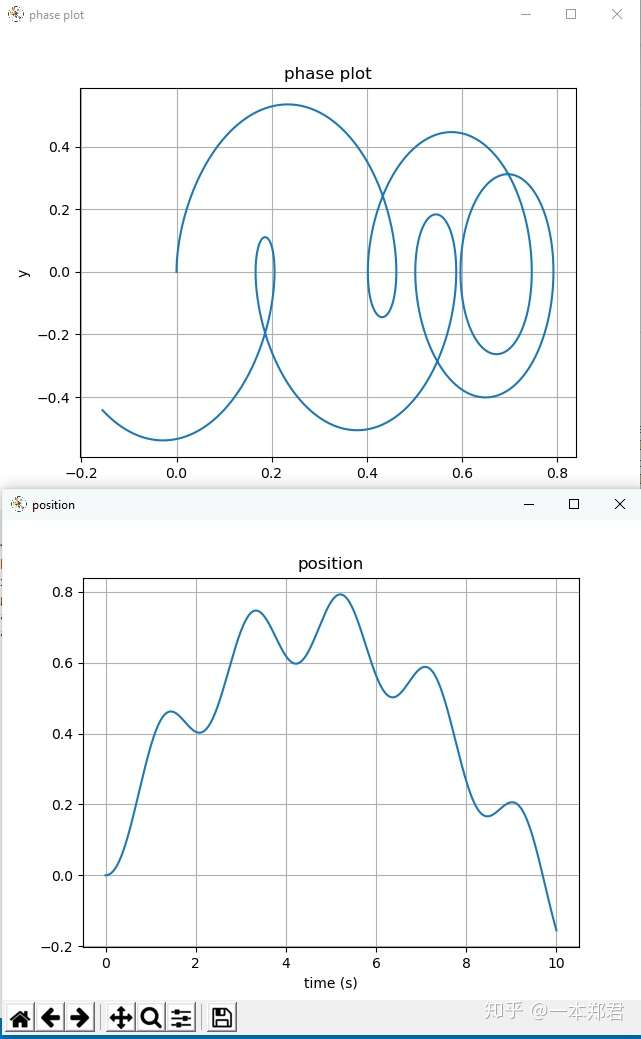## Junruoyu-Zheng / LigralC#MIT

Explore and code with more than 5 million developers，Free private repositories ！：）
Notice: Creating folder will generate an empty file .keep, because not support in Git

# Ligral## 语法

### 节点与连接

`Node | Node[node1]`

`Gain{value:1}`

`node1 -> Gain{value:1} -> Integrator;`

`(Constant{value:1}, Constant{value:2}) -> Calculator{type:'+'};`

Chain list中每一项均可以是一个连接语句，但不需要以“`;`”结尾，如下所示：

```(
Constant{value:1} -> Gain{value:2},
Constant{value:-2} -> Abs
) -> Calculator{type:'+'};```

```node1 -> ThresholdSwitch[switch]:condition;
1 -> switch:first;
2 -> switch:second;```

### 定义语句

```let a = 1;
let vec = [1, 2]
let mat = [-a, 2; vec];```

```route NodeName(parameters;in_ports;out_ports)
some_chains;
end```

```# parameters:
param1, param2:Node, param3=2```

`route NodeName:Gain(parameters;input;output)...`

### 引用

`import module;`

```using module;
module.MyNode -> Scope;```

### 自动装箱

```let a = 1;
a -> Print;```

```let a = [1, 2];
a -> Print;
Constant{value:a} -> Print;```

### 运算符

```let a = 1;

a + 1 -> Print;
(a, 1) -> Calculate{type:'+'} -> Print;

-a -> Print;
(0, a) -> Calculate{type:'-'} -> Print;```

### 矩阵构建解构器

```let vec = [1, -2];
vec -> [Node, Abs] -> Print;
vec -> VSplit -> Split -> (Node, Abs) -> HStack -> VStack -> Print;```

```let mat = [1, 2];
[mat; 2*mat] -> [_, _; Print, _];```

### 设置语句

```conf step_size = 0.01;
conf stop_time = 10;
conf variable_step = false;
conf output_folder = 'output';```

## 用法

`ligral path/file.lig`

-o, --output 输出重定向至给定文件夹
-d, --doc 展示指定节点的文档
-D, --docs 展示所有节点文档
-h 展示示例程序
-s, --size 设置仿真步长
-t, --time 设置仿真时长
--help 展示帮助
--version 展示版本信息

## 示例

```route MassSpringDamper(m,k,d,x0,v0; F; x,v)
F-k*x-d*v -> Gain{value:1/m} -> Integrator{initial:v0} -> v;
v -> Integrator{initial:x0} -> x;
end

SineWave[F]{ampl:10, omega:pi, phi:pi/4};
MassSpringDamper[sys]{m:10, k:1, d:0.1, x0:0, v0: 0};

F -> sys;
sys:x -> Scope{name:'position'};
(sys:x, sys:v) -> PhaseDiagram{name:'phase plot'};

conf step_size = 0.001;
conf stop_time = 10;```